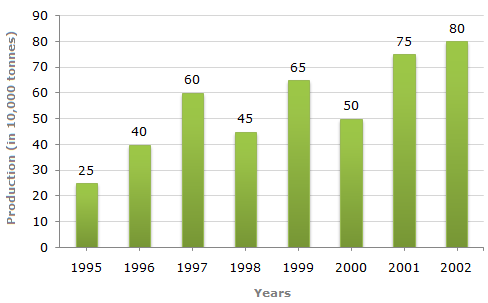# Data Interpretation - Bar Charts - Discussion

Study the bar chart and answer the question based on it.

Production of Fertilizers by a Company (in 1000 tonnes) Over the Years5.

In how many of the given years was the production of fertilizers more than the average production of the given years?

 [A]. 1 [B]. 2 [C]. 3 [D]. 4

Explanation:

Average production (in 10000 tonnes) over the given years

 = 1 (25 + 40 + 60 + 45 + 65 + 50 + 75 + 80) = 55. 8The productions during the years 1997, 1999, 2001 and 2002 are more than the average production.

 Sparsh Chandra said: (Mar 11, 2015) Its written average production of the given years. Never ever written average production directly it should follow year by year average production. So answer should be 3.

 Ani said: (Oct 10, 2018) 1/8 (25+40+60+45+65+50+75+80) = 60. So, 3 is the answer.

 Sreeth said: (Jan 16, 2019) Why 1/8? Please explain.

 Muskan said: (Apr 15, 2019) 1/8 because there is 8 years given.